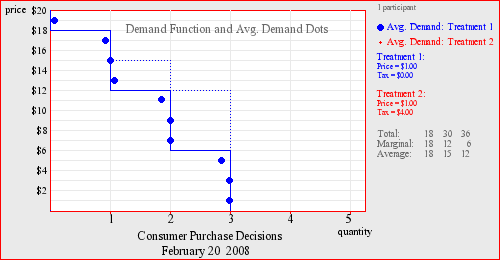### Vecon Lab Demand and Marginal Value

This exercise introduces the notion of demand as a function with "steps" that represent maximum willingness to pay, i.e. the marginal value of consumption. Step function graphs facilitate a subsequent understanding of buyers' surplus, since the area under demand can be calculated visually. (An alternative "employer" context, puts participant plays the role of a firm that must decide how many input units to demand; this framework makes it easier for students to understand where the buyer's values come from, i.e. as added revenues from increasing production.) The exercise is designed to highlight the importance of making decisions at the margin, since purchase decisions based on comparisions of average values and purchase price will lead to lower earnings. One treatment option involves adding a tax to be paid for each unit purchased, which can lead to a discussion of demand shifts. Learning is enhanced by feedback about relative earnings.Key Concepts:DemandMarginal ValueAverage ValueDemand Shift